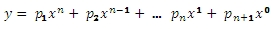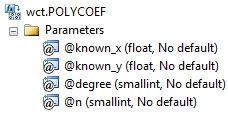# SQL Server polynomial coefficient

POLYCOEF

Updated: 30 April 2011

Use POLYCOEF for obtaining a specific coefficient value from an approximating polynomial for a set of x- and y-values. The coefficients are of a polynomial p(x) of degree n that fits the x- and y-values supplied to the function. The function calculates n+1 polynomial coefficients in descending powers:SyntaxArguments
@known_x
the x-values to be used in the interpolation calculation. @known_x must be of the type float or of a type that implicitly converts to float.
@known_y
the y-values to be used in the interpolation calculation. @known_y must be of the type float or of a type that implicitly converts to float.
@degree
an integer specifying the degree of the polynomial. @degree must be of the type smallint or of a type that implicitly converts to smallint.
@n
the coefficient value to be returned. @n must be of the type smallint or of a type that implicitly converts to smallint.
Return Types
float
Remarks
·         The x- and y-values are passed to the function as pairs
·         If x is NULL or y is NULL, the pair is not used in the calculation.
·         @n must be less than or equal to the number of x-y rows in the GROUP
·         @degree must be less than or equal to the number of x-y rows in the GROUP
·         You can also use the POLYFIT or POLYFIT_q functions to get the coefficients.
·         @degree must remain invariant for the GROUP.
·         @new_x must remain invariant for the GROUP.
·         Available in XLeratorDB / math 2008 only
Examples
In this example, we will use the westclintech SERIESFLOAT function to generate a series of x-values equally spaced in the interval [0, 2.5] and then evaluate the error function, ERF, at those points. We will specify an approximating polynomial of 6 degrees. We will then select the first coefficient
SET NOCOUNT ON

SELECT wct.POLYCOEF(n.x, n.y, 6, 1) as POLYCOEF
FROM (
SELECT SeriesValue as x
,westclintech.wct.ERF(SeriesValue) as y
FROM wct.SeriesFloat(0,2.5,0.1,NULL,NULL)
) n
This produces the following result.
POLYCOEF
----------------------
0.00841937176047103

This is exactly the same result as we would get from the POLYFIT_q function if we had entered the following SQL:
SET NOCOUNT ON

SELECT coe_val
FROM wct.POLYFIT_q('SELECT SeriesValue as x
,westclintech.wct.ERF(SeriesValue) as y
FROM wct.SeriesFloat(0,2.5,0.1,NULL,NULL)'
,6)
where coe_num = 1

This produces the following result.
coe_val
----------------------
0.00841937176047103

If we wanted to obtain all the coefficients in the 6 degree approximating polynomial, we could use the following SQL.
SET NOCOUNT ON

SELECT a.seriesvalue
,wct.POLYCOEF(n.x, n.y, 6, a.SeriesValue) as POLYCOEF
FROM (
SELECT SeriesValue as x
,westclintech.wct.ERF(SeriesValue) as y
FROM wct.SeriesFloat(0,2.5,0.1,NULL,NULL)
) n, wct.SeriesINT(1,7,NULL,NULL,NULL) a
GROUP BY a.SeriesValue
ORDER BY 1
This produces the following result.
seriesvalue               POLYCOEF
----------- ----------------------
1    0.00841937176047103
2    -0.0982995751931375
3      0.421736169319562
4     -0.743462848525445
5      0.147104056213985
6       1.10644604471276
7   0.000441173957975313

This is identical to the result we would have obtained using the POLYFIT_q table-valued function.
SET NOCOUNT ON

SELECT *
FROM wct.POLYFIT_q('SELECT SeriesValue as x
,westclintech.wct.ERF(SeriesValue) as y
FROM wct.SeriesFloat(0,2.5,0.1,NULL,NULL)'
,6)
order by coe_num
This produces the following result.
coe_num                coe_val
----------- ----------------------
1    0.00841937176047103
2    -0.0982995751931375
3      0.421736169319562
4     -0.743462848525445
5      0.147104056213985
6       1.10644604471276

7   0.000441173957975313

### SupportCopyright 2008-2021 Westclintech LLC         Privacy Policy        Terms of Service